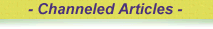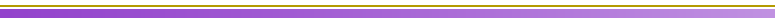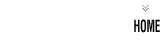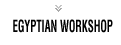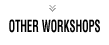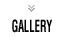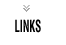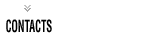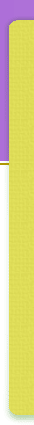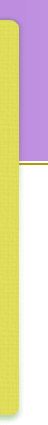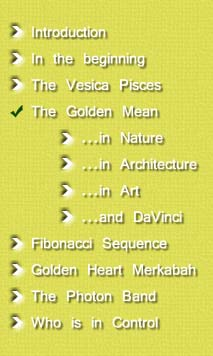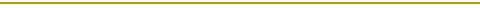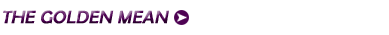Luca Pacioli (also written as Paccioli) wrote a book called De Divina Proportione ( The Divine Proportion ) in 1509. It contains drawings made by Leonardo da Vinci of the 5 Platonic solids. It was probably Leonardo (da Vinci) who first called it the sectio aurea (Latin for the golden section ). Today, some mathematicians use phi (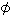) for the golden ratio as on these web pages and others use the Greek letters alpha (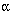) or tau (), the initial letter of tome which is the Greek work for "cut". The Golden mean is equal to 1 2 ( 5 + 1 ), and is the solution of the quadratic equation f 2 - f - 1 = 0. It has many interesting properties. It has often been believed that things such as pictures with dimensions divided in the ratio 1: f are somehow more 'pleasing' or 'aesthetic' than other divisions. Other properties of are that f 2 = f + 1 = 2.618033988... and 1 f = f - 1 = 0.618033988...   In the Fibonacci sequence 1, 1, 2, 3, 5, 8, 13, ..., where each number is the sum of the two previous numbers, the ratio between successive numbers tends to f. Thursday January 16, 2003 The Guardian Think of any two numbers. Make a third by adding the first and second, a fourth by adding the second and third, and so on. When you have written down about 20 numbers, calculate the ratio of the last to the second from last. The answer should be close to 1.6180339887... What's the significance of this number? It's the "golden ratio" and, arguably, it crops up in more places in art, music and so on than any number except pi. Claude Debussy used it explicitly in his music and Le Corbusier in his architecture. There are claims the number was used by Leonardo da Vinci in the painting of the Mona Lisa, by the Greeks in building the Parthenon and by ancient Egyptians in the construction of the Great Pyramid of Khufu. What makes the golden ratio special is the number of mathematical properties it possesses. The golden ratio is the only number whose square can be produced simply by adding 1 and whose reciprocal by subtracting 1. If you take a golden rectangle - one whose length-to-breadth is in the golden ratio - and snip out a square, what remains is another, smaller golden rectangle. The golden ratio is also difficult to pin down: it's the most difficult to express as any kind of fraction and its digits - 10 million of which were computed in 1996 - never repeat. It was this elusive nature that led the 15th-century Italian friar and mathematician Luca Pacioli to equate the golden ratio with the incomprehensibility of God. Although Euclid defined it around 300 BC, and the followers of Pythagoras probably knew of it two centuries earlier, it was Pacioli's three-volume treatise, The Divine Proportion, that was crucial in disseminating the golden ratio beyond the world of mathematics. Da Vinci was a friend of Pacioli's and almost certainly would have read the book, hence the claim that he painted the face of the Mona Lisa to fit inside a hypothetical golden rectangle.